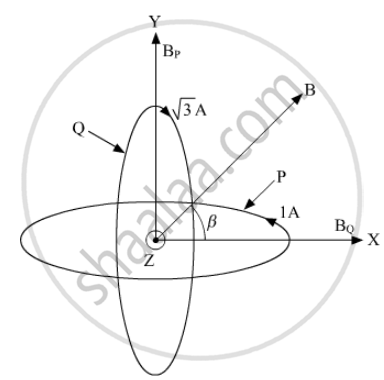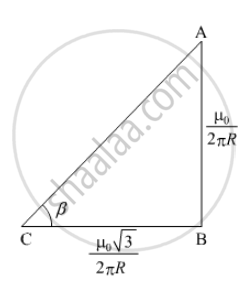PUC Karnataka Science Class 12Department of Pre-University Education, Karnataka
Share

# Two identical circular coils, P and Q each of radius R, carrying currents 1 A and √3A respectively, are placed concentrically and perpendicular to each other lying in the XY and YZ planes. Find the magnitude and direction of the net magnetic field at the centre of the coils. - PUC Karnataka Science Class 12 - Physics

ConceptMagnetic Field on the Axis of a Circular Current Loop

#### Question

Two identical circular coils, P and Q each of radius R, carrying currents 1 A and √3A respectively, are placed concentrically and perpendicular to each other lying in the XY and YZ planes. Find the magnitude and direction of the net magnetic field at the centre of the coils.

#### SolutionMagentic field at the centre of the coil are perpendicular to each other. Therefore net magnetic field (B) is the resultant of the two fields caused due to coil P and Q, respectively.

B_P = (μ_oI)/(2πR)= (μ_o)/(2πR)

B_Q= (μ_oI)/(2πR)= (μ_osqrt3)/(2πR)

Net magnetic field, B = sqrt(B_P^2 + B_Q^2)

=sqrt((μ_o/(2πR))^2 + ( (μ_osqrt3)/(2πR))^2)

= μ_o/(2πR)sqrt4

= μ_o/(πR)

For direction of net magnetic fieldtanβ =(AB)/(BC)

= (μ_o/(2πR))/((μ_osqrt3)/(2πR)) = 1/sqrt3

β= 30^@

The direction of net magnetic field is 30° with the X-direction.

Is there an error in this question or solution?

#### APPEARS IN

Solution Two identical circular coils, P and Q each of radius R, carrying currents 1 A and √3A respectively, are placed concentrically and perpendicular to each other lying in the XY and YZ planes. Find the magnitude and direction of the net magnetic field at the centre of the coils. Concept: Magnetic Field on the Axis of a Circular Current Loop.
S